# 可视化正则表达式教程

github 地址：github.com/qq449245884…

NLP (Natural Language Processing) 是人工智能（AI）的一个子领域。

## 心智模型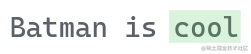``````'cool'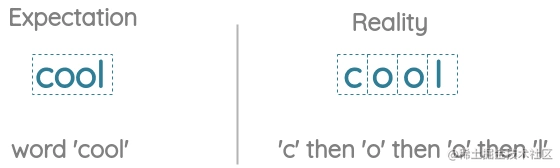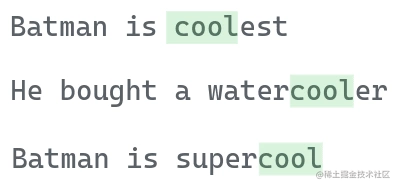## 基本构建基块

#### 特定字符

``````'a'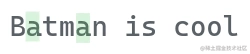``````'3'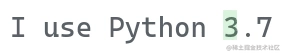``````'A'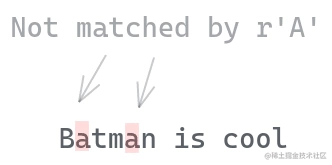#### 空格字符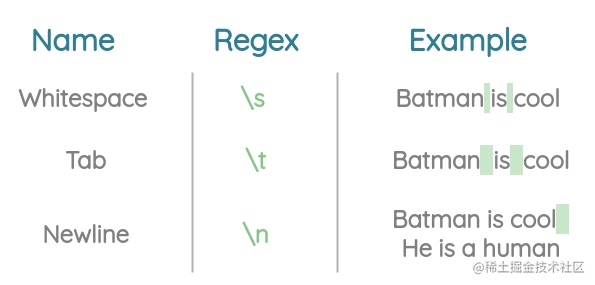• `\r` 回车
• `\f` 换页
• `\e` 可执行模式

#### 特殊字符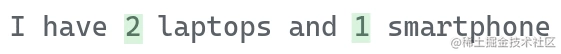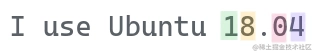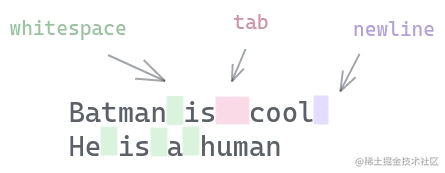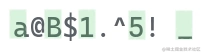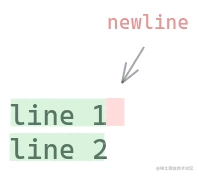``````let str = 'line 1\nline2'
str.match(/./g)

// 结果：["l", "i", "n", "e", " ", "1", "l", "i", "n", "e", "2"]#### 字符集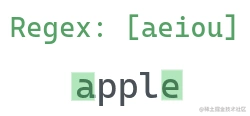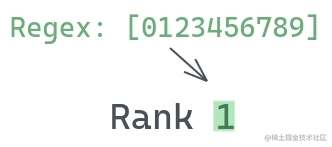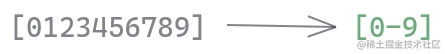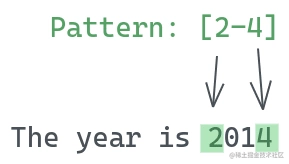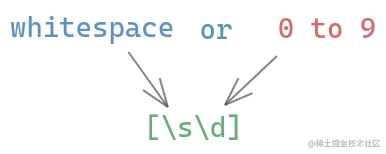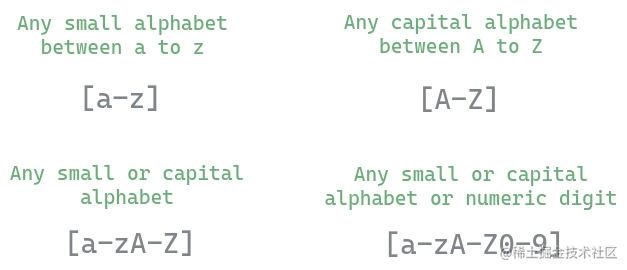#### 锚点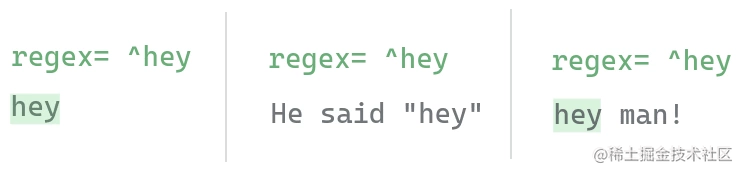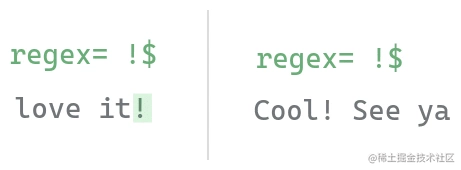#### 转义元字符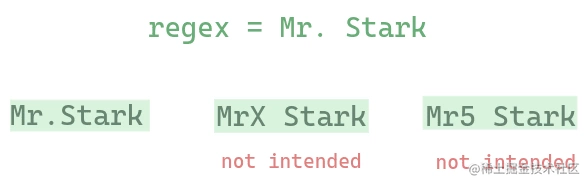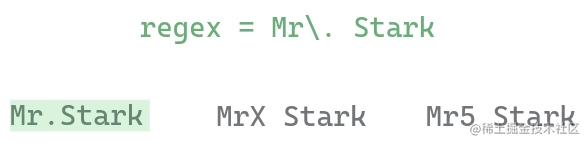``````^ \$ . * + ? { } [ ] \ | ( )

## 重复类

#### 笨的方式来匹配重复字符

``````\d\d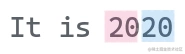#### 量词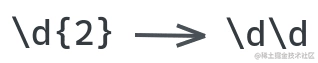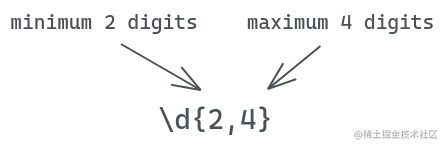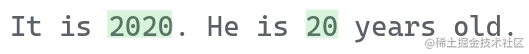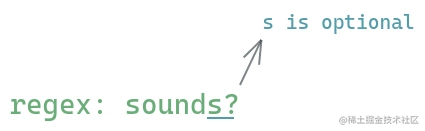`?` 字符表示匹配 0 次或者 一次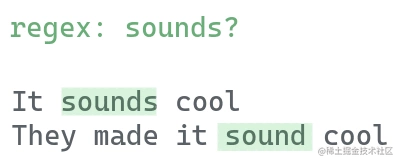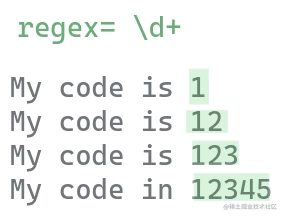`+` 字符表示匹配 一 次或者多次`*` 字符表示匹配 0 次或者多次

## 在Python中的用法

Python在标准库中提供了一个名为`"re"`的模块来使用正则表达式。

#### 对原始字符串的需求

``````pattern = r'\d'

``````>>> pattern = '\t'
>>> print(pattern)

``````>>> pattern = r'\t'
>>> print(pattern)
\t

## 使用 re 模块

``````import re

#### 1. re.findall

``````import re
re.findall(r'\d', '123456')

// ['1', '2', '3', '4', '5', '6']

#### 2. re.match

``````import re

match = re.match(r'batman', 'batman is cool')
print(match)

// <re.Match object; span=(0, 6), match='batman'>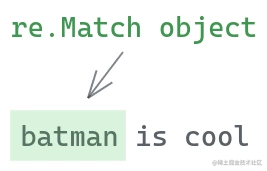``````print(match.group())

// batman

``````import re

match = re.match(r'batman', 'The batman is cool')
print(match)

// None

#### 3. re.search

``````import re

match = re.search(r'batman', 'the batman is cool')
print(match.group())

// batman